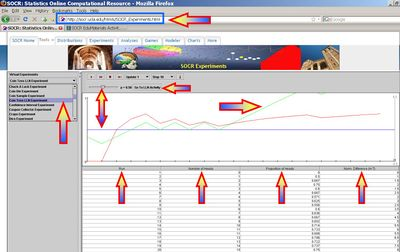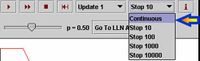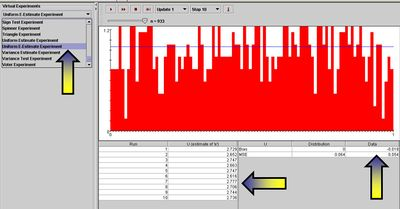# SOCR EduMaterials Activities LawOfLargeNumbers

## Overview

This is part I of a heterogeneous activity that demonstrates the theory and applications of the Law of Large Numbers (LLN). Part II and Part III of this activity contain more examples and diverse experiments. The SOCR LLN applet is available here.

### Goals of the SOCR LLN activity

The goals of this activity are to:

• illustrate the theoretical meaning and practical implications of the LLN;
• present the LLN in varieties of situations;
• provide empirical evidence in support of the LLN-convergence and dispel the common LLN misconceptions.

### Example

The average weight of 10 students from a class of 100 students is most likely closer to the real average weight of all 100 students, compared to the average weight of 3 randomly chosen students from that same class. This is because the sample of 10 is a larger number than the sample of only 3 and better represents the entire class. At the extreme, a sample of 99 of the 100 students will produce a sample average almost exactly the same as the average for all 100 students. On the other extreme, sampling a single student will be an extremely variant estimate of the overall class average weight.

### Statement of the Law of Large Numbers

If an event of probability p is observed repeatedly during independent repetitions, the ratio of the observed frequency of that event to the total number of repetitions converges towards p as the number of repetitions becomes arbitrarily large.

### The theory behind the LLN

Complete details about the weak and strong laws of large numbers may be found here.

## Exercise 1

This exercise illustrates the statement and validity of the LLN in the situation of tossing (biased or fair) coins repeatedly. Suppose we let H and T denote Heads and Tails, the probabilities of observing a Head or a Tail at each trial are $$0<p<1$$ and $$0<1-p<1$$, respectfully. The sample space of this experiment consists of sequences of H's and Ts. For example, an outcome may be $$\{H, H, T, H, H, T, T, T, ....\}$$. If we toss a coin n times, the size of the sample-space is $$2^n$$, as the coin tosses are independent. Binomial Distribution governs the probability of observing $$0\le k\le n$$ Heads in $$n$$ experiments, which is evaluated by the binomial density at $$k$$.

In this case we will be interested in two random variables associated with this process. The first variable will be the proportion of Heads and the second will be the differences of the number of Heads and Tails. This will empirically demonstrate the LLN and its most common misconceptions (presented below). Point your browser to the SOCR Experiments and select the Coin Toss LLN Experiment from the drop-down list of experiments in the top-left panel. This applet consists of a control toolbar on the top followed by a graph panel in the middle and a results table at the bottom. Use the toolbar to flip coins one at a time, 10, 100, 1,000 at a time or continuously! The toolbar also allows you to stop or reset an experiment and select the probability of Heads (p) using the slider. The graph panel in the middle will dynamically plot the values of the two variables of interest (proportion of heads and difference of Heads and Tails). The outcome table at the bottom presents the summaries of all trials of this experiment. From this table, you can copy and paste the summary for further processing using other computational resources (e.g., SOCR Modeler or MS Excel).

• Note: We report the normalized differences of the number of Heads minus the number of Tails in the graph and result table. Let $$H$$ and $$T$$ are the number of Heads and Tails, up to the current trial ($$k$$), respectively. Then we define the normalized difference $$| H - T|$$ = $$p+ ((1-p)H-pT )/(2/3 \times Max_k)$$, where $$Max_k = \max_{1 \le i \le k}{||H-T||_i}$$ and $$||H-T||_i$$ is the maximum difference of Heads and Tails up to the $$i^{th}$$ trial. Observe that the expectation of the normalized difference $$E(|H-T|)=p$$, since $$E((1-p)H-pT)=0$$. This ensures that the normalized differences oscillate around the chosen $$p$$ (the LLN limit of the proportion of Heads) and they are visible within the graph window.Now, select n=100 and p=0.5. The figure below shows a snapshot of the applet. Remember that each time you run the applet the random samples will be different and the figures and results will generally vary. Click on the Run or Step buttons to perform the experiment and observe the proportion of heads and differences evolve over time. Choosing Continuous from the number of experiments drop-down list in the tool bar will run the experiment in a continuous mode (use the Stop button to terminate the experiment in this case). The statement of the LLN in this experiment is simply that as the number of experiments increases the sample proportion of Heads (red curve) will approach the theoretical (user preset) value of p (in this case p=0.5). Try to change the value of p and run the experiment interactively several times. Notice the behavior of the graphs of the two variables we study. Try to pose and answer questions like these:

• If we set p=0.4, how large of a sample-size is needed to ensure that the sample-proportion stays within [0.4; 0.6]?
• What is the behavior of the curve representing the differences of Heads and Tails (red curve)?
• Is the convergence of the sample-proportion to the theoretical proportion (that we preset) dependent on p?
• Remember that the more experiments you run the closer the theoretical and sample proportions will be (by LLN). Go in Continuous run mode and watch the convergence of the sample proportion to $$p$$. Can you explain in words, why can't we expect the second variable of interest (the differences of Heads and Tails) to converge?## Exercise 2

The second SOCR demonstration of the law of large numbers will be quite different and practically useful. Here we show how the LLN implies practical algorithms for estimation of transcendental numbers. The two most popular transcendental numbers are $$\pi$$ and e.

### Estimating e using SOCR simulation

The SOCR E-Estimate Experiment provides the complete details of this simulation. In a nutshell, we can estimate the value of the natural number e using random sampling from Uniform distribution. Suppose $$X_1, X_2, ..., X_n$$ are drawn from uniform distribution on (0, 1) and define $$U= {\operatorname{argmin}}_n { \left (X_1+X_2+...+X_n > 1 \right )}$$, note that all $$X_i \ge 0$$.

Now, the expected value $$E(U) = e \approx 2.7182$$. Therefore, by LLN, taking averages of $$\left \{ U_1, U_2, U_3, ..., U_k \right \}$$ values, each computed from random samples $$X_1, X_2, ..., X_n \sim U(0,1)$$ as described above, will provide a more accurate estimate (as $$k \rightarrow \infty$$) of the natural number e.

The Uniform E-Estimate Experiment, part of SOCR Experiments, provides a hands-on demonstration of how the LLN facilitates stochastic simulation-based estimation of e.### Estimating $$\pi$$ using SOCR simulation

Similarly, one may approximate the transcendental number $$\pi$$, using the SOCR Buffon’s Needle Experiment. Here, the LLN again provides the foundation for a better approximation of $$\pi$$ by virtually dropping needles (many times) on a tiled surface and observing if the needle crosses a tile grid-line. For a tile grid of size 1, the odds of a needle-line intersection are $${ 2 \over \pi} \approx 0.63662$$. In practice, to estimate $$\pi$$ from a number of needle drops (N), we take the reciprocal of the sample odds-of-intersection.

## Experiment 3

Suppose we row 10 loaded hexagonal (6-face) dice 8 times and we are interested in the probability of observing the event A={3 ones, 3 twos, 2 threes, and 2 fours}. Assume the dice are loaded to the small outcomes according to the following probabilities of the 6 outcomes (one is the most likely and six is the least likely outcome).

 x 1 2 3 4 5 6 P(X=x) 0.286 0.238 0.19 0.143 0.095 0.048
P(A)=?

Of course, we can compute this number exactly as:

$P(A) = {10! \over 3!\times 3! \times 2! \times 2! } \times 0.286^3 \times 0.238^3\times 0.19^2 \times 0.143^2 = 0.00586690138260962656816896.$

However, we can also find a pretty close empirically-driven estimate using the SOCR Dice Experiment.

For instance, running the SOCR Dice Experiment 1,000 times with number of dice n=10, and the loading probabilities listed above, we get an output like the one shown below.Now, we can actually count how many of these 1,000 trials generated the event A as an outcome. In one such experiment of 1,000 trials, there were 8 outcomes of the type {3 ones, 3 twos, 2 threes and 2 fours}. Therefore, the relative proportion of these outcomes to 1,000 will give us a fairly accurate estimate of the exact probability we computed above $P(A) \approx {8 \over 1,000}=0.008$.

Note that that this approximation is close to the exact answer above. By the Law of Large Numbers, we know that this SOCR empirical approximation to the exact multinomial probability of interest will significantly improve as we increase the number of trials in this experiment to 10,000.

## Hands-on activities

The following practice problems will help students experiment with the SOCR LLN activity and understand the meaning, ramifications and limitations of the LLN.

• Run the SOCR Coin Toss LLN Experiment twice with stop=100 and p=0.5. This corresponds to flipping a fair coin 100 times and observing the behavior of the proportion of heads across (discrete) time.
• What will be different in the outcomes of the 2 experiments?
• What properties of the 2 outcomes will be very similar?
• If we did this 10 times, what is expected to vary and what may be predicted accurately?
• Use the SOCR Uniform e-Estimate Experiment to obtain stochastic estimates of the natural number $$e \approx 2.7182$$.
• Try to explain in words, and support your argument with data/results from this simulation, why is the expected value of the variable U (defined above) equal to e, $$E(U)=e$$.
• How does the LLN come into play in this experiment?
• How would you go about in practice if you had to estimate $$e^2 \approx 7.38861124$$ ?
• Similarly, try to estimate $$\pi \approx 3.141592$$ and $$\pi^2 \approx 9.8696044$$ using the SOCR Buffon’s Needle Experiment.
• Run the SOCR Roulette Experiment and bet on 1-18 (out of the 38 possible numbers/outcomes).
• What is the probability of success (p)?
• What does the LLN imply about p and repeated runs of this experiment?
• Run this experiment 3 times. What is the sample estimate of p ($$\hat{p}$$)? What is the difference $$p-\hat{p}$$? Would this difference change if we ran the experiment 10 or 100 times? How?
• In 100 Roulette experiments, what can you say about the difference of the number of successes (outcome in 1-18) and the number of failures? How about the proportion of successes?

## Common Misconceptions regarding the LLN

• Misconception 1: If we observe a streak of 10 consecutive heads (when p=0.5, say) the odds of the $$11^{th}$$ trial being a Head is > p! This is of course, incorrect, as the coin tosses are independent trials (an example of a memoryless process).
• Misconception 2: If run large number of coin tosses, the number of heads and number of tails become more and more equal. This is incorrect, as the LLN only guarantees that the sample proportion of heads will converge to the true population proportion (the p parameter that we selected). In fact, the difference |Heads - Tails| diverges!